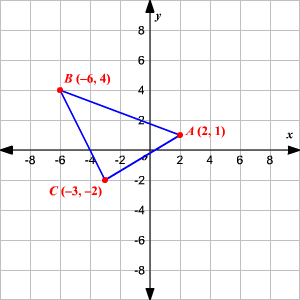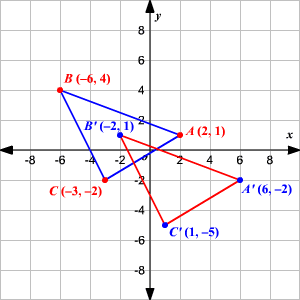# Translations

A translation is a transformation that occurs when a figure is moved from one location to another location without changing its size, shape or orientation. In the coordinate plane we can draw the translation if we know the direction and how far the figure should be moved.

To translate the point $P\left(x,y\right)$ , $a$ units right and $b$ units up, use ${P}^{\text{'}}\left(x+a,y+b\right)$ . To translate the point $P\left(x,y\right)$ , $a$ units left and $b$ units down, use ${P}^{\text{'}}\left(x-a,y-b\right)$ .

Example:

Triangle $ABC$ has vertices $A\left(2,1\right),B\left(-6,4\right),\text{\hspace{0.17em}}\text{\hspace{0.17em}}\text{and}\text{\hspace{0.17em}}\text{\hspace{0.17em}}C\left(-3,-2\right)$ . If $\Delta ABC$ is translated $4$ units right and $3$ units down, what are the coordinates of the vertices of the image $\Delta {A}^{\text{'}}{B}^{\text{'}}{C}^{\text{'}}$ ?

Draw the triangle $ABC$ with coordinates $A\left(2,1\right),B\left(-6,4\right),\text{\hspace{0.17em}}\text{\hspace{0.17em}}\text{and}\text{\hspace{0.17em}}\text{\hspace{0.17em}}C\left(-3,-2\right)$ .Now we have to translate the triangle $4$ units right and $3$ units down. So, use the formula,

${P}^{\text{'}}\left(x+4,y-3\right)$

$\begin{array}{l}A\left(2,1\right)\to {A}^{\text{'}}\left(2+4,1-3\right)\to {A}^{\text{'}}\left(6,-2\right)\\ B\left(-6,4\right)\to {B}^{\text{'}}\left(-6+4,4-3\right)\to {B}^{\text{'}}\left(-2,1\right)\\ C\left(-3,-2\right)\to {C}^{\text{'}}\left(-3+4,-2-3\right)\to {C}^{\text{'}}\left(1,-5\right)\end{array}$

To check the answer graph and compare $\Delta ABC$ and its image $\Delta {A}^{\text{'}}{B}^{\text{'}}{C}^{\text{'}}$ . Each image vertex is $4$ units right and $3$ units down from each preimage vertex.Therefore, the coordinates of the image $\Delta {A}^{\text{'}}{B}^{\text{'}}{C}^{\text{'}}$ are ${A}^{\text{'}}\left(6,-2\right),{B}^{\text{'}}\left(-2,1\right),\text{\hspace{0.17em}}\text{\hspace{0.17em}}\text{and}\text{\hspace{0.17em}}\text{\hspace{0.17em}}{C}^{\text{'}}\left(1,-5\right)$ .